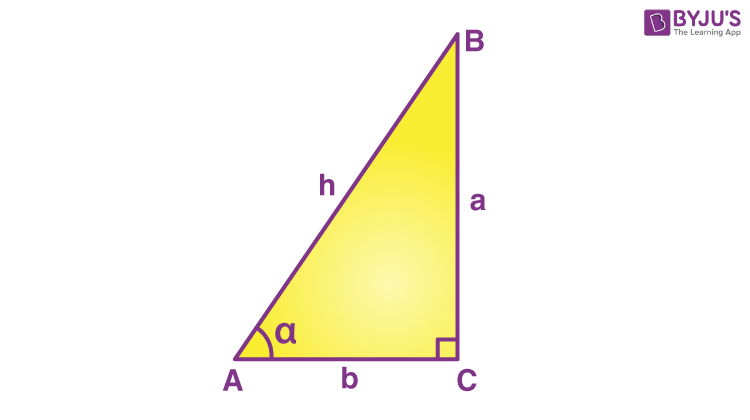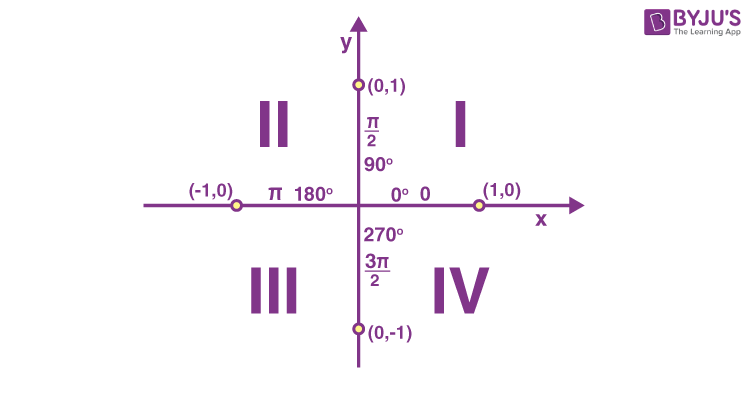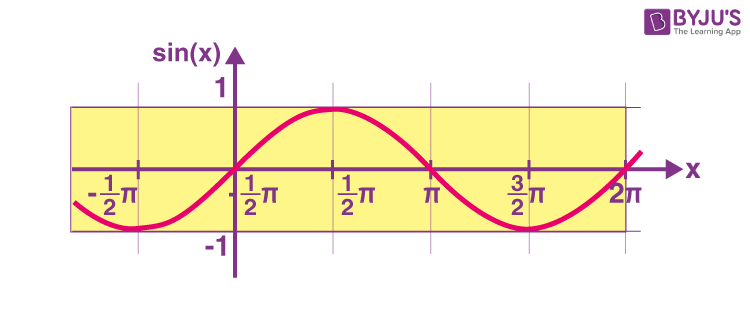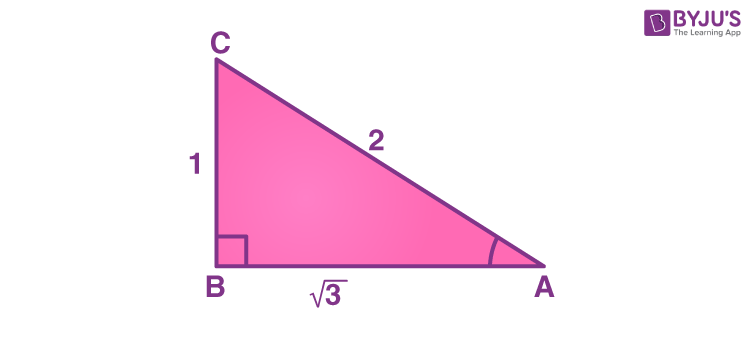# Sine Function

Sine function is one of the three primary functions in trigonometry, the others being cosine, and tan functions. The sine x or sine theta can be defined as the ratio of the opposite side of a right triangle to its hypotenuse. Here, a detailed lesson on this trigonometric function i.e. the sine function is given which will include the following topics:

## Sine Function Definition

In trigonometry, the sine function can be defined as the ratio of the length of the opposite side to that of the hypotenuse in a right-angled triangle. The sine function is used to find the unknown angle or sides of a right triangle.

For any right triangle, say ABC, with an angle α, the sine function will be:

Sin α= Opposite/ Hypotenuse

## Sine Function Formula

In a right-angled triangle, the sine of an angle is equal to the ratio of side opposite to the angle (also called perpendicular) and hypotenuse.

Suppose, ‘α’ is the angle, in a right triangle ABC.Then, the sine formula is given by:

Sin α= Opposite side/ Hypotenuse

or

Sin α = Perpendicular/Hypotenuse

As per the given figure, the sine formula becomes,

Sin α = a/h

where ‘a’ is the opposite side to angle ‘α’ and ‘h’ is the hypotenuse.

Other Trigonometric Functions:

## Sine Function Values Table

 Sine Degrees Values Sine 0° 0 Sine 30° 1/2 Sine 45° 1/√2 Sine 60° √3/2 Sine 90° 1 Sine 120° √3/2 Sine 150° 1/2 Sine 180° 0 Sine 270° -1 Sine 360° 0

 Sine 1 Degree is 0.84 Sine 2 Degree is 0.91 Sine 5 Degree is -0.96 Sine 10 Degree is -0.54 Sine 20 Degree is 0.91 Sine 30 Degree is -0.99 Sine 40 Degree is 0.75 Sine 50 Degree is -0.26 Sine 70 Degree is 0.77 Sine 80 Degree is -0.99 Sine 100 Degree is -0.50 Sine 105 Degree is -0.97 Sine 210 Degree is 0.47 Sine 240 Degree is 0.95 Sine 330 Degree is -0.13 Sine 350 Degree is 0.95

## Properties of Sine function as per Quadrants

The sine function has values positive or negative depending upon the quadrants. In the above table, it is seen that sine 270 is negative while sine 90 is positive. For the sine function, the value depends upon the quadrants and is positive for the first and second quadrants while it is negative for values in the third and fourth quadrants.### Sine Function Domain and Range

As we know, the sine function is defined for all real numbers, so the domain of y = sin x is the set of all real numbers, i.e. R. The range of sine function is the closed interval [-1, 1]. That means, -1 ≤ y ≤ 1 or -1 ≤ sin x ≤ 1. However, the range of this function can be given as per the quadrants. Observe the below table to get the range of sine function in different quadrants.

 Degree Range Quadrant Sine Function Sign Sine Value Range 0 to 90 Degrees 1st Quadrant + (Positive) 0 < sin(x) < 1 90 to 180 Degrees 2nd Quadrant + (Positive) 0 < sin(x) < 1 180 to 270 Degrees 3rd Quadrant – (Negative) -1 < sin(x) < 0 270 to 360 Degrees 4th Quadrant – (Negative) -1 < sin(x) < 0

## Sine Function Graph

The sine graph looks like the image given below. The sine graph or sinusoidal graph is an up-down graph and repeats every 360 degrees i.e. at 2π. In the below-given diagram, it can be seen that from 0, the sine graph rises till +1 and then falls back till -1 from where it rises again.

The function y = sin x is an odd function, because;

sin (-x) = -sin x### Sine function Period and Amplitude

From the above, we can observe that if x increases (or decreases) by an integral multiple of 2π, the sine function values do not change. Thus,

sin (2nπ + x) = sin x, n ∈ Z

sin x = 0, if x = 0, ± π, ± 2π , ± 3π, …, i.e., when x is an integral multiple of π

Sometimes, we can also write this as:

sin x = 0 implies x = nπ, where n is an integer

Thus, we can say that the value of sin x repeats after an interval of 2π. Therefore, the period of sin x is 2π.

While sketching a graph of the given sine function, convert the given function to the general form to find the different parameters such as amplitude, phase shift, vertical shift and period.

The general form:

a sin (bx – c) + d

Where,

|a|  = Amplitude

2π/|b| = Period

c/b = Phase shift

d = Vertical shift

For example, the Sine function whose period is 6 is sin(πx/3).

This can be derived as:

y = sin kx

Period of sin kx = 2π/|k|

2π/|k| = 6

|k| = π/3

Thus, y = sin(πx/3)

Similarly, we can calculate the sine function with period 3.

## Inverse Sine Function (arcsine)

The sine inverse function is used to measure the angle of a right-angled triangle from the given ratios. The inverse of sine is denoted as arcsine, asin or sin-1.

Suppose a right triangle is taken with side 1, 2, and √3. Now, to calculate angle a, the sine function can be used as-Now, sine (a) = opposite/hypotenuse i.e ½.

The angle A can now be calculated using the arcsine function.

sin−1(½) = a

This will give the value of angle “a” as 30°

## Sine Function Identities

Some of the common sine identities are:

• sine (θ) = cos(π/2 − θ) = 1/cosec(θ)
• arcsin (sin θ) = θ, for −π/2 ≤ θ ≤ π/2
• cos(θ) + sin(θ) = 1
• Sin (2x) = 2sin (x) cos (x)
• Cos (2x) = cos2(x) − sin2(x)

There are various other sine identities. The values of other trigonometric functions in terms of sine are also given below which are extremely useful for solving various types of questions.

### Other Trigonometric Functions in Terms of Sine Function

 Trigonometric Functions Represented as Sine cos θ $$\begin{array}{l}\pm \sqrt{1\,-\,sin^{2}(\theta)}\end{array}$$ tan θ $$\begin{array}{l}\pm \frac{sin(\theta)}{\sqrt{1\,-\,sin^{2}(\theta)}}\end{array}$$ cot θ $$\begin{array}{l}\pm \frac{\sqrt{1\,-\,sin^{2}(\theta)}}{sin(\theta)}\end{array}$$ sec θ $$\begin{array}{l}\pm \,\frac{1}{\sqrt{1\,-\,sin^{2}(\theta)}}\end{array}$$

## Sine Function in Calculus

For sine function f(x) = sin(x), the derivative and the integral will be given as:

• Derivative of sin(x), f′(x) = cos(x)
• Integral of sin(x), ∫f(x) dx = −cos(x) + C (where C is the constant of integration)

## Law of Sine Functions in Trigonometry

According to law of sines in trigonometry, a relation is established between the sides a, b, and c and angles opposite to those sides A, B and C for an arbitary triangle. The relation is as follows.In the above diagram, A, B, C are the angles while a, b, c are the lengths of the sides. Now, according to sine law,

$$\begin{array}{l}\frac{a}{Sin\,A}\,=\,\frac{b}{Sin\,B}\,=\,\frac{c}{Sin\,C}\,=\,d\end{array}$$

(Here, d is the diameter of the triangle’s circumcircle).

Check out the law of sines article to know more about it and to know the proof of the law of sine, examples, and other details.

Also Check: Law of Cosine

## Sine Functions Worksheet

1. What is the sine of 60?
2. What is sin 270?
3. What is sin 120 degrees?
4. Evaluate sin 360 degrees.
5. Derive the value of sin 60 geometrically.
6. Find the value of sin 30 degree geometrically and prove sin 30 geometrically.
7. Why sin 90 is 1?
8. What is sine divided by cosine?
9. An aeroplane is flying at 6000 feet above the ground. At what angle should the plane descend to land on the target runway if it is 10,000 feet away from the runway?
10. In a triangle ABC, AC is 14 cm, CB is 10 cm, and angle CBA is 63 degrees. Calculate angle CAB and the length of AB.
11. For a triangle ABC, sine (ABC) is 0.6 and the length of BC is 12 cm. Find the length of AC (hypotenuse).
12. A 55 m rope connects is connected to a point on the ground from the top of a pole. If the rope makes 60 degree angle to the ground, calculate the height of the pole.

## Frequently Asked Questions – FAQs

### What is a sine function in trigonometry?

In trigonometry, a sine function of an angle is the ratio of the opposite side (perpendicular) and hypotenuse of a right triangle.

### Is sine function even or odd?

The sine function is an odd function whereas cosine is an even function.

### What is the sine function formula?

According to sine function, sine formula is given by:
Sin A = Perpendicular/Hypotenuse
where perpendicular is the opposite side of angle A.

### What is the value of sin 0?

Sine of zero degrees angle is equal to 0.

### Other Trigonometry Related Topics

Stay tuned with BYJU’S and get more trigonometry and maths-related articles in a simple and detailed way. Also, register at BYJU’S to get access to 1000+ hours of engaging video lessons for different subjects and classes.

Quiz on Sine Function